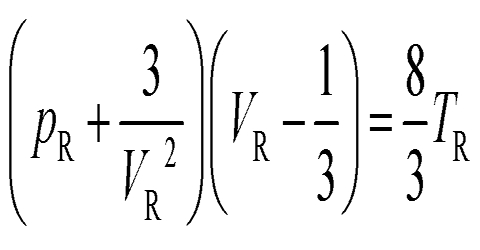# Equation of the Corresponding States

The Equation of the Corresponding States

Using the values of a from equation

3Vc = (b + RTc/Pc); 3Vc2 = (a/Pc); Vc3 = (ab/Pc);

b from equation Vc = 3b; Pc = a/27b2; Tc = 8a/27Rb and

R from equation: RTc/PcVc = 8/3 = 2.67; in terms of the critical constants the van der Waals’ equation may be written in the form:

[P + (3Vc2 Pc/V2)] (V – Vc/3) = [8/3 (PcVcT/Tc)]

This can be rearranges to the form by dividing by PcVc.

[P/Pc + (3Vc2/V2)] (V/Vc – 1/3) = [8/3 . T/Tc]

If the pressure, volume and temperature of a gas are expressed in terms of their critical values such that;

P/Pc = π; V – Vc = Ø; and T/Tc = θ; then the equation becomes;

(π + 3/Ø2) (Ø – 1/3) = 8/3 θ

or, (π + 3/Ø2) 3(Ø – 1) = 8θ … … … (1)

Here π is called the reduced pressure, Ø the reduced volume and θ the reduced temperature. Equation (1) is called the reduced equation of state or the equation of corresponding states.

The important thing about this equates is that it does not contain any constant which is peculiar to the individual gas; therefore, it should be capable of describing all gases. In this respect the reduced equation is perfectly general unlike van der Waals equation which has the constants, a and b; both depending on the individual gas. Otherwise, the reduced equation has the same properties as the van der Waals equation, e.g., it has three roots and represents the behavior of substances both in the liquid and gaseous states.

If two or more substances are at the same reduced temperature and under the same reduced pressure then according to equation (1) their reduced volumes should also be equal. When these conditions are fulfilled the substances are said to be in corresponding states and the above statement is taken as the expression for the law of corresponding stales. For many substances the law is in agreement with experimental results but there are also considerable discrepancies, particularly in the case of alcohols etc. In fact the reduced equation of state has been found to have the same limitations as the van der Waals equation from which it is derived.